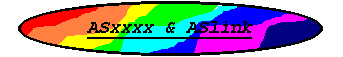## ASZ8 Assembler#### ```Z8 REGISTER SET The following is a list of the Z8 registers used by ASZ8: r0 ... r15 - 8-bit accumulators rr0 ... rr15 - 16-bit accumulators Z8 INSTRUCTION SET The following tables list all Z8 mnemonics recognized by the ASZ8 assembler. The designation [] refers to a required ad- dressing mode argument. The following list specifies the format for each addressing mode supported by ASZ8: #data immediate byte data addr location/branch address r0 ... r15 8-bit registers rr0 ... rr15 16-bit registers @rn or register indirect addressing (rn) @rrn or register indirect addressing (rrn) @addr or indirect addressing (addr) offset(rn) indexed register addressing The terms data, addr, and offset may all be expressions. The designation CC refers to a condition code argument. The following table contains all the valid condition codes supported by ASZ8: f Always False - t Always True - c Carry C=1 nc No Carry C=0 z Zero Z=1 nz Non-Zero Z=0 pl Plus S=0 mi Minus S=1 ov Overflow V=1 nov No Overflow V=0 eq Equal Z=1 ne Not Equal Z=0 ge Greater Than or Equal (S XOR V)=0 lt Less Than (S XOR V)=1 gt Greater Than (Z OR (S XOR V))=0 le Less Than or Equal (Z OR (S XOR V))=1 uge Unsigned ge C=0 ult Unsigned lt C=1 ugt Unsigned gt (C=0 AND Z=0)=1 ule Unsigned le (C OR Z)=1 Note that not all addressing modes are valid with every instruc- tion, refer to the Z8 technical data for valid modes. Load Instructions clr [] ld [],[] ldc [],[] pop [] push [] Arithmetic Instructions adc [],[] add [],[] cp [],[] da [] dec [] decw [] inc [] incw [] sbc [],[] sub [],[] Logical Instructions and [],[] com [] or [],[] xor [],[] Program Control Instructions call [] djnz [],[] iret jp CC,[] jr CC,[] ret Bit Manipulation Instructions tcm [],[] tm [],[] and [],[] or [],[] xor [],[] Block Transfer Instructions ldci [],[] Rotate and Shift Instructions rl [] rlc [] rr [] rrc [] sra [] swap [] Cpu Control Instructions ccf di ei halt nop rcf scf srp [] stop wait ```

##### Go to the Documentation Index